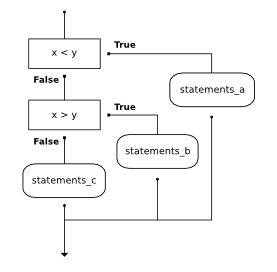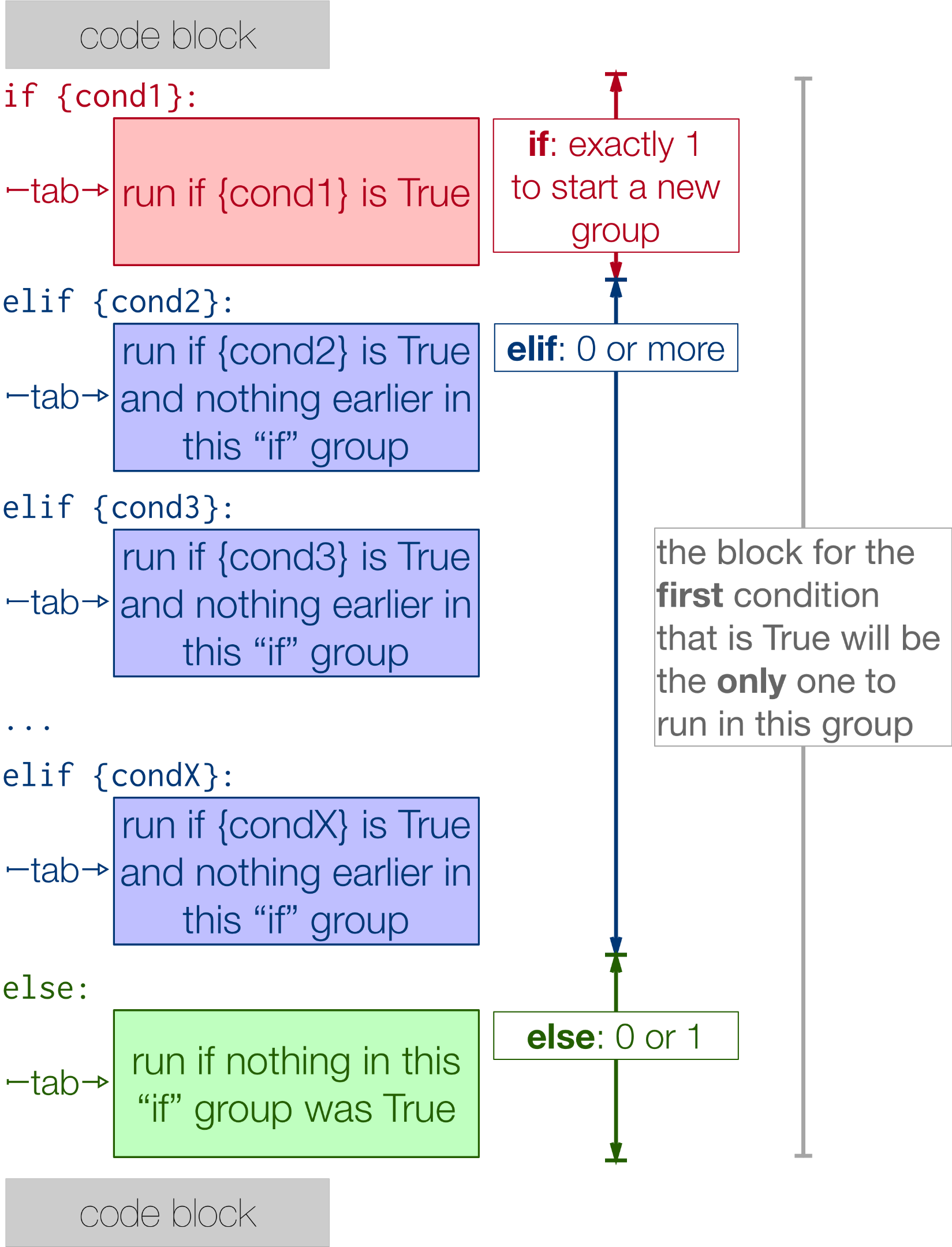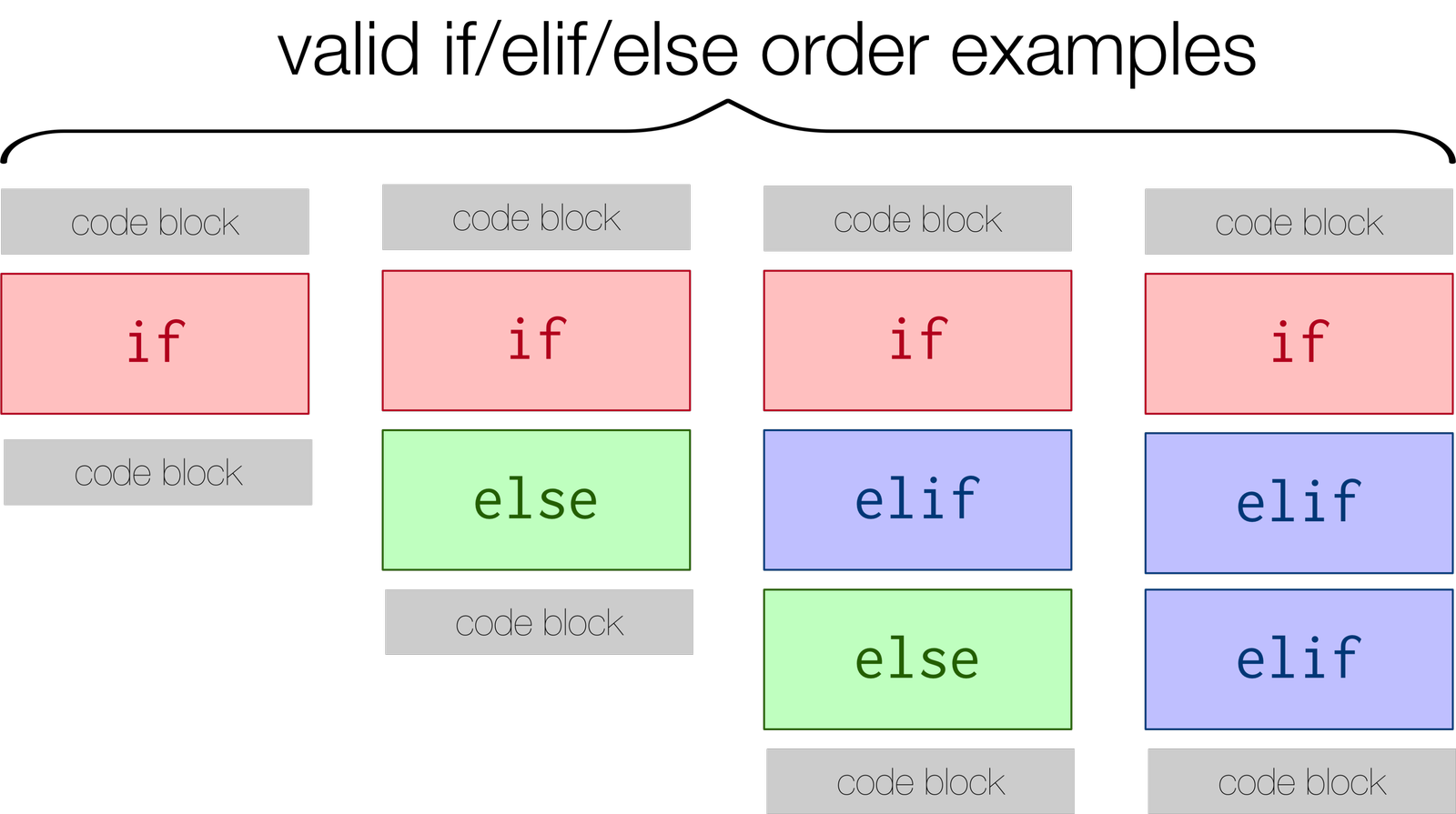# 8.9. Chained conditionals¶

Python provides an alternative way to write nested selection such as the one shown in the previous section. This is sometimes referred to as a chained conditional.

if x < y:
print("x is less than y")
elif x > y:
print("x is greater than y")
else:
print("x and y must be equal")


The flow of control can be drawn in a different orientation but the resulting pattern is identical to the one shown above.elif is an abbreviation of else if. Again, exactly one branch will be executed. There is no limit of the number of elif statements but only a single (and optional) final else statement is allowed and it must be the last branch in the statement.Each condition is checked in order. If the first is false, the next is checked, and so on. If one of them is true, the corresponding branch executes, and the statement ends. Even if more than one condition is true, only the first true branch executes.

Here is the same program using elif.

The following image highlights different kinds of valid conditionals that can be used. Though there are other versions of conditionals that Python can understand (imagine an if statement with twenty elif statements), those other versions must follow the same order as seen below.Which of I, II, and III below gives the same result as the following nested if?

# nested if-else statement
x = -10
if x < 0:
print("The negative number ",  x, " is not valid here.")
else:
if x > 0:
print(x, " is a positive number")
else:
print(x, " is 0")

I.

if x < 0:
print("The negative number ",  x, " is not valid here.")
else (x > 0):
print(x, " is a positive number")
else:
print(x, " is 0")

II.

if x < 0:
print("The negative number ",  x, " is not valid here.")
elif (x > 0):
print(x, " is a positive number")
else:
print(x, " is 0")

III.

if x < 0:
print("The negative number ",  x, " is not valid here.")
if (x > 0):
print(x, " is a positive number")
else:
print(x, " is 0")

• I only
• You can not use a Boolean expression after an else.
• II only
• Yes, II will give the same result.
• III only
• No, III will not give the same result. The first if statement will be true, but the second will be false, so the else part will execute.
• II and III
• No, Although II is correct III will not give the same result. Try it.
• I, II, and III
• No, in I you can not have a Boolean expression after an else.

What will the following code print if x = 3, y = 5, and z = 2?

if x < y and x < z:
print("a")
elif y < x and y < z:
print("b")
else:
print("c")

• a
• While the value in x is less than the value in y (3 is less than 5) it is not less than the value in z (3 is not less than 2).
• b
• The value in y is not less than the value in x (5 is not less than 3).
• c
• Since the first two Boolean expressions are false the else will be executed.

Create one conditional to find whether “false” is in string str1. If so, assign variable output the string “False. You aren’t you?”. Check to see if “true” is in string str1 and if it is then assign “True! You are you!” to the variable output. If neither are in str1, assign “Neither true nor false!” to output.

Create an empty list called resps. Using the list percent_rain, for each percent, if it is above 90, add the string ‘Bring an umbrella.’ to resps, otherwise if it is above 80, add the string ‘Good for the flowers?’ to resps, otherwise if it is above 50, add the string ‘Watch out for clouds!’ to resps, otherwise, add the string ‘Nice day!’ to resps. Note: if you’re sure you’ve got the problem right but it doesn’t pass, then check that you’ve matched up the strings exactly.

We have created conditionals for you to use. Do not change the provided conditional statements. Find an integer value for x that will cause output to hold the values True and None. (Drawing diagrams or flow charts for yourself may help!)